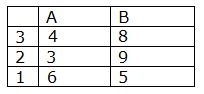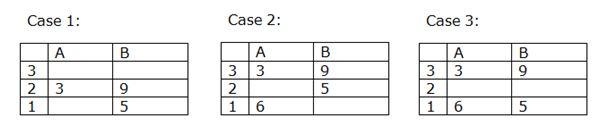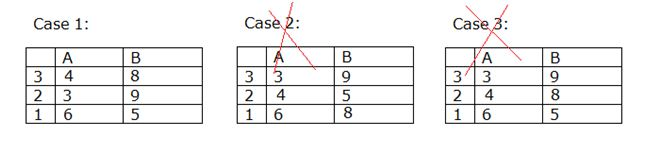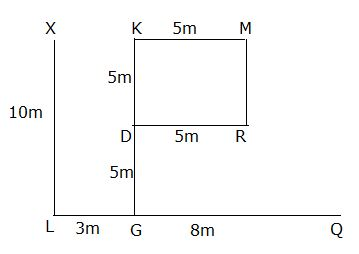# IBPS RRB PO Mains Reasoning Ability Questions 2019 (Day-03)

Dear Aspirants, Our IBPS Guide team is providing new series of Reasoning Questions for IBPS RRB PO Mains 2019 so the aspirants can practice it on a daily basis. These questions are framed by our skilled experts after understanding your needs thoroughly. Aspirants can practice these new series questions daily to familiarize with the exact exam pattern and make your preparation effective.

Check here for IBPS RRB PO Mains Mock Test 2019

Check here for IBPS RRB Clerk Mock Test 2019

Check here for IBPS PO Exam Mock Test 2019

### Click Here to Subscribe Crack High Level Puzzles & Seating Arrangement Questions PDF 2019 Plan

[WpProQuiz 6824]

Directions (1-4): There are two rows given and to find out the resultant of a particular row we need to follow the following steps: –

Step 1: If an odd number is followed by an even number (but not a cube number) then the resultant comes by multiplying the numbers.

Step 2: If an odd number is followed by a cube number then the resultant will be the difference of the cube number and the odd number.

Step 3: If an odd number is followed by another odd number (but not a cube or square) then the resultant will be the addition of both the numbers.

Step 4: If an even number is followed by an odd (non-prime) number then the resultant will be the difference of the odd number and the even number.

Step 5: If an even number is followed by an odd (prime) number then the resultant will be the division of higher number by lower number.

1) Find the difference between the resultant of the first and second row.

121    2    11

63     8    23

a) 53

b) 110

c) 97

d) 56

e) None of these

2) Find the product of the resultant of the given two rows.

80   9    37

78   13  15

a) 872

b) 972

c) 1024

d) 876

e) None of these

3) Find the sum of the resultant of the given two rows.

63   43   9

85   16   5

a) 266

b) 397

c) 280

d) 369

e) None of these

4) What is the resultant of the second row, if X is the resultant of the first row?

17                     512                              13

X-3        2X-50% of 9x+132-20              729

a) 853

b) 897

c) 907

d) 895

e) None of these

Directions (5-8): Study the following information carefully and answer the questions given below.

There are three floors in a given building such that floor 1 is below floor 2 and floor 2 is below floor 3. In the building there are two flats named A and B in each floor. Flat B is to the east of Flat A. In the building each flat has an area of 1728ft and there are certain numbers of rooms and no two flats have the same number of rooms. Total number of rooms on floor number 3 is twice the number of rooms in flat A of floor number 1. The flat in which the area of each room is 576 ft is to the west of the flat in which the area of each room is 192 ft. Number of rooms in the flat which is above 5 rooms is 192 ft. There is no flat to the east of the flat whose room’s area is 216 ft. The flat in which the area of each room is 432 ft is above the flat in which the area of each room is 288 ft. Number of rooms in flat B of floor 2 is equal to sum of the rooms in flat A of floors 1and 2.

5) What is the total number of rooms on floor number 1?

a) 10

b) 15

c) 9

d) 11

e) None of these

6) What is the area of each room in flat B of floor number 1?

a) 288 ft

b) 216 ft

c) 334.5 ft

d) 345.6 ft

e) None of these

7) How many rooms are there to the east of the flat in which the area of each room is 432 ft?

a) 5

b) 8

c) 6

d) 7

e) None of these

8) How many rooms are in the flat that is just below the flat A of floor number 3?

a) 9

b) 4

c) 6

d) 8

e) None of these

Directions (9-10): Study the following information carefully and answer the questions given below.

Point X is 10m north of Point L. Point G is 10m south of Point K. Point M is 5m east of Point K. Point M is 5mnorth of Point R. Point D is 5m west of Point R. Point L is 11m west of Point Q. Point G is 3m east of Point L.

9) What is the position of point L with respect to point M?

a) South East

b) North West

c) South West

d) North East

e) None of these

10) If Point H is south of Point M and east of Point S, Point S is 4m north of L, then what is the distance between Point H and Point S?

a) 5m

b) 3m

c) 4m

d) 8m

e) None of these

Directions (1-4):

121   2   11=>57*6=242 (by step 1)

242 11=>242/11=22 (by step 5)

63   8   23=>63-8=55 (by step 2)

55+23=>78 (by step 3)

78-22=56

Hence d is the correct answer.

80   9   37=>80-9=>71 (by step 4)

71+37=>108 (by step3)

78   13   15=>78/13=>6 (by step 5)

15-6=>9 (by step 4)

108*9=972

Hence b is the correct answer.

63   43   9=>63+43=>106 (by step3)

106-9=>97 (by step 4)

85  16   5=>85*16=>1360 (by step 1)

1360/5=272 (by step 3)

Hence d is the correct answer.

17  512  13=>512-17=>495 (by step 2)

495+13=>508 (by step 3)

508-3        2(508)-50% of 9(508)+132-20               729

505           1121         729=>505+1121=>1626(by step 1)

1626   729=>1626-729=>897 (by step 2)

Hence b is the correct answer.

Directions (5-8):Total number of rooms on floor number 3 is twice the number of rooms in flat A of floor number 1. The flat in which the area of each room is 576 ft is to the west of the flat in which the area of each room is 192 ft. Number of rooms in the flat which is above 5 rooms is 192 ft.

1728/576=3 rooms

1728/192=9 roomsThere is no flat to the east of the flat whose room’s area is 216ft. The flat in which the area of each room is 432 ft is above the flat in which the area of each room is 288 ft. Number of rooms in flat B of floor 2 is equal to sum of the rooms in flat A of floors 1 and 2.

1728/216=8 rooms

1728/432=4 rooms

1728/288=6 roomsCase 2 and 3 will be dropped because number of rooms in flat B of floor 2 is equal to sum of the rooms in flat A of floors 1 and 2.

Clearly, Total number of rooms on floor 1 = 6+5=11

Hence d is the correct answer.

Clearly, Area of each room in flat B of floor number 1 =1728/5= 345.6 ft.

Hence d is the correct answer.

Clearly, The room’s area of flat A of floor 1= 432 ft.

Number of rooms present to the east of the flat in which the room’s area is 432 ft=8

Hence b is the correct answer.

Number of rooms in the flat that is just below the flat A of floor number 3=3

Hence, e is the correct answer.

Directions (9-10):Clearly, Point L is South-west of M

LM = √(100+64)= 2√41